Home Practice
For learners and parents For teachers and schools
Textbooks
Full catalogue
Pricing SupportLog in

We think you are located in United States. Is this correct?

A quadrilateral is a four-sided polygon. A quadrilateral has four straight sides and four vertices. Different quadrilaterals have different angle and side relationships. The figure below shows five different types of quadrilaterals:

• A: Square
• B: Rectangle
• C: Parallelogram
• D: Rhombus
• E: Trapezium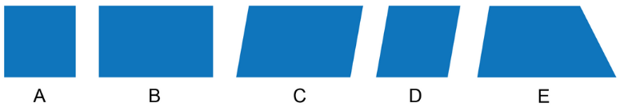a four-sided polygon

A quadrilateral can be divided into two triangles by drawing a diagonal from one vertex to the opposite vertex. As a result, we can deduce that the sum of the interior angles of a quadrilateral is equal to twice the sum of the interior angles of a triangle:

$\text{Sum of angles of quadrilateral} = 2 \times 180^{\circ} = 360^{\circ}$### Parallelograms

A parallelogram is a quadrilateral with both pairs of opposite sides equal and parallel.

The diagram shows parallelogram $$ABCD$$:

• Opposite sides are equal: $$AB = DC$$ and $$BC = AD$$.
• Opposite sides are parallel: $$AB \parallel DC$$ and $$BC \parallel AD$$.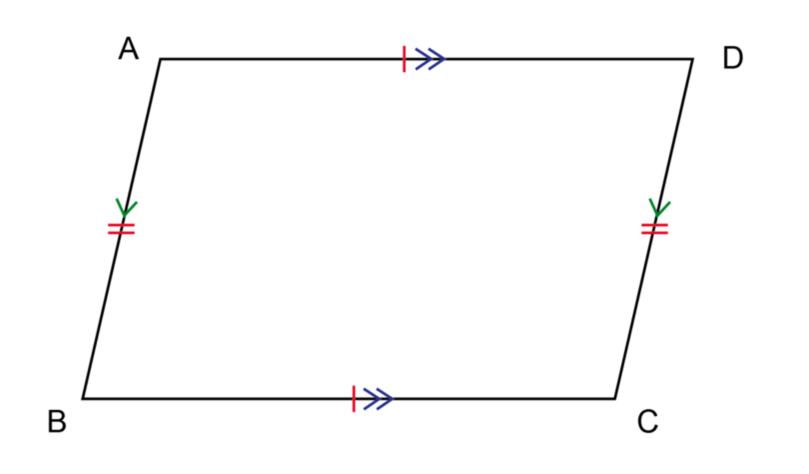A parallelogram has four interior angles. A special property of the interior angles of parallelogram is that the interior opposite angles are equal. A parallelogram has two pairs of opposite angles that are equal.

The diagram shows parallelogram $$PQRS$$ with opposite angles equal: $$\hat{P} = \hat{R}$$ and $$\hat{Q} = \hat{S}$$.A quadrilateral can be classified as a parallelogram if any of the following properties are true:

• Both pairs of opposite sides are parallel.
• Both pairs of opposite angles are equal.
• One pair of opposite sides is equal and parallel.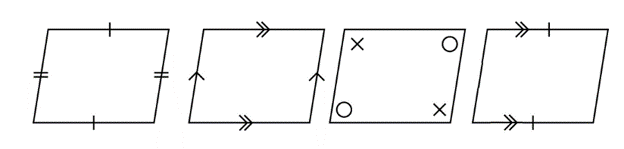## Worked Example 11.7: Calculating angles and sides of a parallelogram

$$QRST$$ is a parallelogram with $$\hat{Q} = 54^{\circ}$$, $$\hat{R} = 126^{\circ}$$, $$QT = 9 \text{ units}$$, $$RS = 9 \text{ units}$$ and $$TS = 5 \text{ units}$$. Find the values of $$w$$, $$x$$ and $$y$$.### Use the properties of a parallelogram to find the unknown values.

In parallelogram $$QRST$$:

\begin{align} \hat{Q} &= \hat{S}\ (\text{opp. } \angle\text{s equal}) \\ \hat{Q} &= y \\ &= 54^{\circ} \end{align} \begin{align} \hat{R} &= \hat{T}\ (\text{opp. } \angle\text{s equal}) \\ \hat{R} &= x \\ &= 126^{\circ} \end{align} \begin{align} QR &= TS\ (\text{opp. sides equal}) \\ QR &= w \\ &= 5 \text{ units} \end{align}

In parallelogram $$QRST$$, $$y = 54^{\circ}$$, $$x = 126^{\circ}$$ and $$w = 5 \text{ units}$$.

temp text

### Rectangles

A rectangle is quadrilateral with opposites equal and parallel and interior angles equal to $$90^{\circ}$$.rectangle
a quadrilateral with opposites equal and parallel and interior angles equal to $$90^{\circ}$$

The diagonal of a rectangle divides the rectangle into two right-angled triangles. We can use the theorem of Pythagoras to calculate the length of unknown sides of these triangles. You will learn more about this theorem in Chapter 15.A quadrilateral can be classified as a rectangle if any of the following properties are true:

• All interior angles are equal to $$90^{\circ}$$.
• Both pairs of opposite sides are equal and one interior angle is $$90^{\circ}$$.
• Both pairs of opposite sides are parallel and one interior angle is $$90^{\circ}$$.
• One pair of opposite sides is equal and parallel and one interior angle is $$90^{\circ}$$.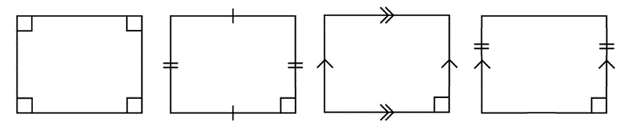## Worked Example 11.8: Calculating angles and sides of a rectangle

$$MNPQ$$ is a rectangle with $$\hat{N} = 90^{\circ}$$ and $$M\hat{P}N = 59^{\circ}$$.

Find the values of $$a$$, $$b$$, $$c$$ and $$d$$.### Use the properties of a rectangle to find the unknown angles.

In rectangle $$MNPQ$$:

\begin{align} \hat{Q} &= \hat{N} = 90^{\circ}\ (\text{opp. } \angle\text{s equal}) \\ \hat{Q} &= a = 90^{\circ} \end{align}

In rectangle $$MNPQ$$:

\begin{align} \hat{M} &= \hat{P} = 90^{\circ}\ (\text{opp. } \angle\text{s equal}) \\ b + 59^{\circ} &= 90^{\circ}\ (\text{right angle of rectangle}) \\ b &= 90^{\circ} − 59^{\circ} \\ b &= 31^{\circ} \end{align}

In $$\triangle MNP$$:

\begin{align} d + 90^{\circ} + 59^{\circ} &= 180^{\circ} (\angle \text{ sum of } \triangle) \\ d &= 180^{\circ} − 149^{\circ} \\ d &= 31^{\circ} \end{align}

In rectangle $$MNPQ$$:

\begin{align} c + d &= 90^{\circ}\ (\text{opp. } \angle\text{s equal}) \\ c &= 90^{\circ} − 31^{\circ} \\ c &= 59^{\circ} \end{align}

In rectangle $$MNPQ$$, $$a = 90^{\circ}$$, $$b = 31^{\circ}$$, $$c = 59^{\circ}$$ and $$d = 31^{\circ}$$.

temp text

### Squares

A square is parallelogram with all sides equal and interior angles equal to $$90^{\circ}$$.A diagonal divides the square into two right-angled isosceles triangles. We can use the theorem of Pythagoras to calculate the length of unknown sides of these triangles.A quadrilateral can be classified as a square if any of the following properties are true:

• All sides are equal and one interior angle is $$90^{\circ}$$.
• One pair of adjacent sides is equal and all interior angles are $$90^{\circ}$$.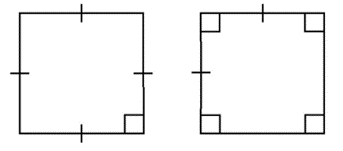## Worked Example 11.9: Calculating angles and sides of a square

$$ABCD$$ is a square with $$\hat{D} = 3t$$, $$AB = s$$ and $$AD = 7 \text{ cm}$$.

Find the values of $$s$$ and $$t$$.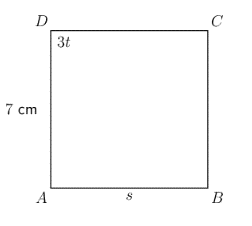### Use the properties of a square to find the unknown values.

In square $$ABCD$$:

\begin{align} \hat{D} &= 3t = 90^{\circ}\ (\text{right angle of square}) \\ 3t &= 90^{\circ} \\ \frac{3t}{3} &= \frac{90^{\circ}}{3} \\ t &= 30^{\circ} \end{align}

In square $$ABCD$$:

\begin{align} AD &= AB = s\ (\text{equal sides of square}) \\ AD &= 7 \text{ cm} \\ \therefore AB &= s = 7 \text{ cm} \end{align}

In square $$ABCD$$, $$t = 30^{\circ}$$ and $$s = 7 \text{ cm}$$

temp text

### Rhombus

A rhombus is a quadrilateral with both pairs of opposite sides parallel and all sides equal.

rhombus
a quadrilateral with both pairs of opposite sides parallel and all sides equal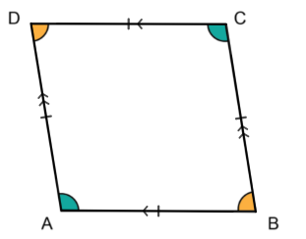A rhombus has two pairs of opposite angles that are equal. A diagonal divides the rhombus into two isosceles triangles.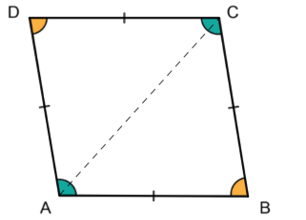A quadrilateral can be classified as a rhombus if any of the following properties are true:

• All sides are equal.
• Both pairs of opposite sides are parallel and one pair of adjacent sides is equal.
• Both pairs of opposite angles are equal and one pair of adjacent sides is equal.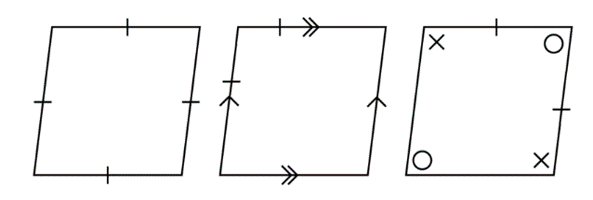## Worked Example 11.10: Calculating angles and sides of a rhombus

$$EFGH$$ is a rhombus with $$\hat{H} = x + 40^{\circ}$$, $$\hat{F} = 102^{\circ}$$, $$FE= w$$ and $$FG = 10 \text{ m}$$. Find the values of $$x$$ and $$w$$.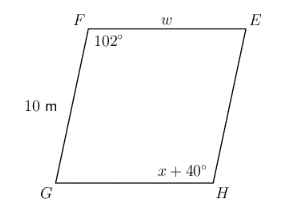### Use the properties of a rhombus to find the unknown values.

In rhombus $$EFGH$$:

\begin{align} \hat{F} &= \hat{H} = 102^{\circ}\ (\text{opp. } \angle\text{s equal}) \\ \hat{H} &= x + 40^{\circ} = 102^{\circ} \\ x &= 102^{\circ} − 40^{\circ} \\ \therefore x &= 62^{\circ} \end{align}

In rhombus $$EFGH$$:

\begin{align} FG &= FE = w\ (\text{equal sides of rhombus}) \\ FG &= 10 \text{ m} \\ \therefore w &= 10 \text{ m} \end{align}

In rhombus $$EFGH$$, $$x = 62^{\circ}$$ and $$w = 10 \text{ m}$$

temp text

### Trapeziums

A trapezium is a quadrilateral with one pair of opposite sides parallel.

trapezium
a quadrilateral with one pair of opposite sides parallel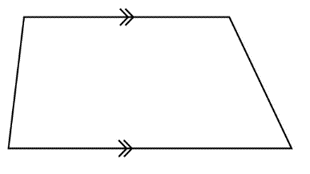An isosceles trapezium has one pair of opposite sides parallel and the other pair of opposite sides equal. The base angles of an isosceles trapezium are equal.## Worked Example 11.11: Calculating angles of a trapezium

$$CDEF$$ is a trapezium with $$\hat{C} = 82^{\circ}$$, $$\hat{E} = 142^{\circ}$$, $$\hat{F} = 98^{\circ}$$ and $$\hat{D} = 2p$$. Determine the value of $$p$$.### Use the properties of a trapezium to find the unknown value.

In trapezium $$CDEF$$:

\begin{align} \hat{C} + \hat{D} + \hat{E} +\hat{F} &= 360^{\circ}\ (\text{sum of } \angle\text{s quad.}) \\ 82^{\circ} + 2p + 142^{\circ} + 98^{\circ} &= 360^{\circ} \\ 2p &= 360^{\circ} − 322^{\circ} \\ 2p &= 38^{\circ} \\ \therefore p &= 19^{\circ} \end{align}

In trapezium $$CDEF$$, $$p = 19^{\circ}$$.

### Kites

A kite is a quadrilateral with two pairs of adjacent sides equal. In kite $$ABCD$$, the two shorter adjacent sides are equal, $$AD = CD$$, and the two longer adjacent sides are equal, $$AB = CB$$.

kite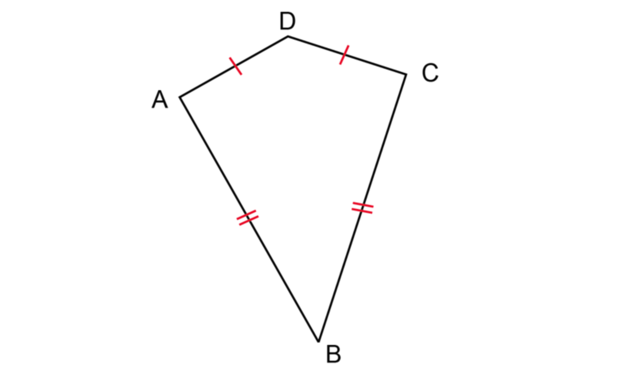There is one pair of opposite angles that are equal.## Worked Example 11.12: Calculating angles of a kite

$$ABCD$$ is a kite with $$\hat{A} = 2z + 16^{\circ}$$, $$\hat{D} = 103^{\circ}$$, $$\hat{C} = z$$, $$AD = AB$$ and $$CD = CB$$. Find the values of $$z$$.### Use the properties of a kite to find the unknown value.

In kite $$ABCD$$:

\begin{align} \hat{D} &= \hat{B} = 103^{\circ}\ (\text{opp. } \angle\text{s equal}) \\ \hat{A} + \hat{B} + \hat{C} + \hat{D} &= 360^{\circ}\ (\text{sum of } \angle\text{s quad.}) \\ 2z + 16^{\circ} + 103^{\circ} + z + 103^{\circ} &= 360^{\circ} \\ 3z &= 360^{\circ} − 222^{\circ} \\ 3z &= 138^{\circ} \\ \therefore z &= 46^{\circ} \end{align}

In kite $$ABCD$$, $$z = 46^{\circ}$$.

Exercise 11.2

Identify each shape.

1.2.3.4.5.1. Trapezium
2. Trapezium
3. Kite
4. Trapezium
5. Kite

Use the diagram to answer these questions.

1. Name the type of quadrilateral.
2. Determine the value of $$t$$.1. Trapezium
2. $t = 70^{\circ}$

Given: trapezium $$ABCD$$ with $$AD \parallel BC$$, $$\hat{A} = 86^{\circ}, \hat{B} = 150^{\circ}$$ and $$\hat{D} = 94^{\circ}$$. Find $$\hat{C}$$.

$\hat{C} = 30^{\circ}$

Calculate the value of $$x$$.$x = 100^{\circ}$

In kite $$ABCD$$, $$AB = AD$$ and $$BC = DC$$. $$\hat{A} = 110^{\circ}, \hat{C} = 50^{\circ}$$ and $$\hat{D} = \hat{B} = y$$. Determine the value of $$y$$.$y = 100^{\circ}$

Kite $$MNPQ$$ has $$MN = NP$$ and $$PQ = QM$$. $$\hat{M} = 105^{\circ}$$, $$\hat{P} = 105^{\circ}$$ and $$\hat{Q} = 67^{\circ}$$. Find $$\hat{N}$$.

$\hat{N} = 83^{\circ}$